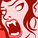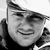# QlikView App Dev

Discussion Board for collaboration related to QlikView App Development.

Announcements
Support Cases coming to Qlik Community Oct. 4! Start chats, open cases, explore resources: READ DETAILS
cancel
Showing results for
Did you mean:Contributor

## calculate average of variable in qlikview

I want to calculate average of sum of all projects but it's giving me nested aggregation error. I have tried using total but it's giving me 0 as a result.

=Num(sum({<year_month_num = {\$(=Max(year_month_num))}>}aggr(\$(=[Overall Step Weightage Formula]),Project,Month)),'#0.##%')

6 RepliesMVP

You will get nested aggregation errors when trying to do an aggregation inside an aggregation (without using aggr() in between. Example: sum({\$} avg())

Right now your expression looks like this

Num(Sum({} aggr( %unknown%)))

As long as %unknown% is a normal aggregation such as sum(), max () or avg() then your expression setup should be fine and you should not get that nested aggregation warning.

So please check your inner dollar expanded expression to see if the error lies there.

Plees ekskuse my Swenglish and or Norweglish spelling misstakesContributor
Author

Below Expressions are giving me error. Can we apply average directly on variable?

(sum(Avg({<year_month_num = {\$(=Max(year_month_num))}>}aggr(\$(=[Overall Step Weightage Formula]),Month))))*100

or

(sum(Avg({<year_month_num = {\$(=Max(year_month_num))}>}aggr(\$(=[Overall Step Weightage Formula]),Month))))*100MVP

What's the difference between the two?

What is the content of [Overall Step Weightage Formula]?

Plees ekskuse my Swenglish and or Norweglish spelling misstakesContributor
Author

I am attaching sample .qvw file. And secondly I want variable value to calculate on the basis of Measure/Department but this formula only calculating value for Sub-Projects. Please have a lookContributor
Author

.QVW sample file is attached in above comment. Overall Step Weightage Formula is different for each sub-Project. Overall Step Weightage formula is a set expression itself. For Example for Sub-Project NFR-EV charger , Overall Step Weightage Formula is

num(
sum(aggr(RangeSum(Above(TOTAL IF([Progress Description] <> ' ',If(Sum(Aggr(If(Match([Progress Description], [Sub Activities]), 1, 0), [Progress Description],[Sub Activities])) > 0,[Sub Activities Weightage]*1,0),0) , 0, RowNo(TOTAL))),[Sub-Project], Month,[Fiscal Year]))
,'0.##%')Contributor
Author

@Vegar Please look into the attached .qvw file.Community Browser